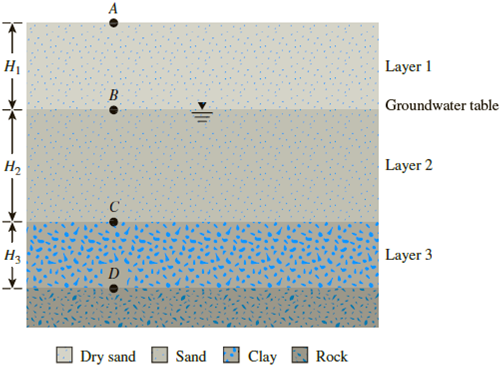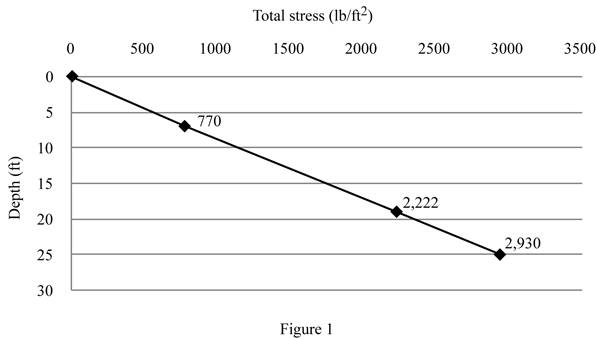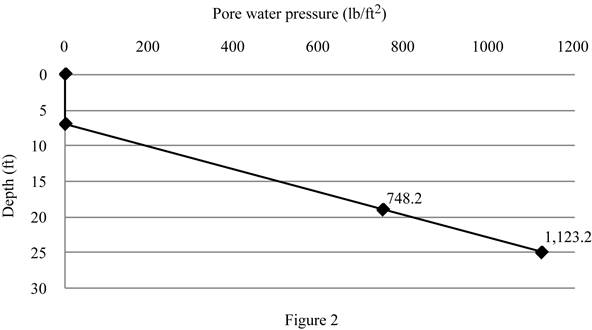Chapter 9, Problem 9.1PPrinciples of Geotechnical Enginee...

9th Edition
Braja M. Das + 1 other
ISBN: 9781305970939

Solutions

Chapter
SectionPrinciples of Geotechnical Enginee...

9th Edition
Braja M. Das + 1 other
ISBN: 9781305970939
Textbook Problem

9.1 Through 9.3 A soil profile consisting of three layers is shown in Figure 9.25. Calculate the values of σ, u, and σ′ at points A, B, C, and D for the following cases. In each case, plot the variations of σ, u, and σ′ with depth. Characteristics of layers 1, 2, and 3 for each case are given below:Figure 9.25To determine

Find the total stress σ , pore water pressure u , and effective stress σ at points A, B, C, and D and plot the variations of total stress, pore water pressure, and effective stress.

The total stress at point A is zero_.

The pore water pressure at point A is zero_.

The effective stress at point A is zero_.

The total stress at point B is 770lb/ft2_.

The pore water pressure at point B is zero_.

The effective stress at point B is 770lb/ft2_.

The total stress at point C is 2,222lb/ft2_.

The pore water pressure at point C is 748.8lb/ft2_.

The effective stress at point C is 1,473.2lb/ft2_.

The total stress at point D is 2,930lb/ft2_.

The pore water pressure at point D is 1,123.2lb/ft2_.

The effective stress at point D is 1,806.8lb/ft2_.

Explanation

Given information:

The thickness H1 of soil layer 1 is 7 ft.

The thickness H2 of soil layer 2 is 12 ft.

The thickness H3 of soil layer 3 is 6 ft.

The dry unit weight γd of soil in the first layer is 110lb/ft3.

The saturated unit weight γsat of soil in the second layer is 121lb/ft3.

The saturated unit weight γsat of soil in the third layer is 118lb/ft3.

Calculation:

Calculate the total stress at point A (0 ft).

σ=0

Thus, the total stress at point A is zero_.

Calculate the pore water pressure at point A (0 ft).

u=0

Thus, pore water pressure at point A is zero_.

Calculate the effective stress at point A (0 ft) using the relation.

σ=σu

Substitute 0 for σ and 0 for u.

σ=00=0

Thus, effective stress at point A is zero_.

Calculate the total stress at point B (7 ft) using the relation.

σ=γd×H1

Substitute 110lb/ft3 for γd and 7 ft for H1.

σ=110×7.0=770lb/ft2

Thus, total stress at point B is 770lb/ft2_.

Calculate the pore water at point B (7 ft) using the relation.

u=0

Thus, the pore water pressure at point B is zero_.

Calculate the effective stress at point B (7 ft) using the relation.

σ=σu

Substitute 770lb/ft2 for σ and 0 for u.

σ=7700=770lb/ft2

Thus, the effective stress at point B is 770lb/ft2_.

Calculate the total stress at point C (19 ft) using the relation.

σ=γd×H1+γsat×H2

Substitute 110lb/ft3 for γd, 7 ft for H1, 121lb/ft3 for γsat, and 12 ft for H2.

σ=110×7.0+121×12=2,222lb/ft2

Thus, the total stress at point C is 2,222lb/ft2_.

Calculate the pore water pressure at point C (19 ft) using the relation.

u=γw×H2

Here, γw is the unit weight of the water.

Take the unit weight of the water as 62.4lb/ft3.

Substitute 62.4lb/ft3 for γw and 12 ft for H2.

u=62.4×12=748.8lb/ft2

Thus, the pore water pressure at point C is 748.8lb/ft2_.

Calculate the effective stress at point C (19 ft) using the relation.

σ=σu

Substitute 2,222lb/ft2 for σ and 748.8lb/ft2 for u.

σ=2,222748.8=1,473.2lb/ft2

Thus, the effective stress at point C is 1,473.2lb/ft2_.

Calculate the total stress at point D (25 ft) using the relation.

σ=γd×H1+γsat×H2+γsat×H3

Substitute 110lb/ft3 for γd, 7 ft for H1, 121lb/ft3 for γsat, 12 ft for H2, 118lb/ft3 for γsat, and 6 ft for H3.

σ=110×7.0+121×12+118×6=2,930lb/ft2

Thus, the total stress at point D is 2,930lb/ft2_.

Calculate the pore water pressure at point D (25 ft) using the relation.

u=γw×(H2+H3)

Substitute 62.4lb/ft3 for γw, 12 ft for H2, and 6 ft for H3.

u=62.4×(12+6)=1,123.2lb/ft2

Thus, the pore water pressure at point D is 1,123.2lb/ft2_.

Calculate the effective stress at point D (25 ft) using the relation.

σ=σu

Substitute 2,930lb/ft2 for σ and 1,123.2lb/ft2 for u.

σ=2,9301,123.2=1,806.8lb/ft2

Thus, the effective stress at point D is 1,806.8lb/ft2_.

Show the plot between depth and total stress as in Figure 1.Show the plot between depth and pore water pressure as in Figure 2.Show the plot between depth and effective stress as in Figure 3.The Solution to Your Study Problems

Bartleby provides explanations to thousands of textbook problems written by our experts, many with advanced degrees!

Get Started

What is a task? What is an event? What is a milestone?

Systems Analysis and Design (Shelly Cashman Series) (MindTap Course List)

Determine the tension in each of the three ropes supporting the 800-lb crate.

International Edition---engineering Mechanics: Statics, 4th Edition

List some applications that use low-pressure piping.

Welding: Principles and Applications (MindTap Course List)

Define the terms, motherboard, chip, integrated circuit, and transistor.

Enhanced Discovering Computers 2017 (Shelly Cashman Series) (MindTap Course List)

Find all the answers to your study problems with bartleby.
Textbook solutions plus Q&A. Get As ASAP arrow_forward